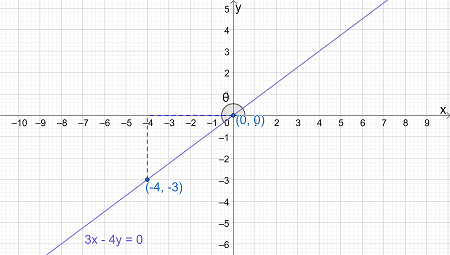# Sketch an angle \theta in standard position such that the terminal side of \theta falls on the...

## Question:

Sketch an angle {eq}\theta {/eq} in standard position such that the terminal side of {eq}\theta {/eq} falls on the line {eq}3x - 4y = 0 {/eq} where {eq}x = -4 {/eq}, and then determine the 6 trigonometric functions for {eq}\theta {/eq} (correctly labeled).

## Sketching Angles by Lines:

While sketching an angle, the standard placement of the base leg is along the positive direction of the {eq}x- {/eq}axis.

The terminal leg of the angle must meet the positive {eq}x- {/eq}axis at {eq}(0,0) {/eq}.

If the terminal leg is determined by a line, there must be a specification on which part of the line is to be considered.

This is because the terminal leg is a ray and a line can be split into two rays.

The angle lies on the line {eq}3x - 4y = 0 {/eq}.

To sketch the angle, plot the line {eq}3x - 4y = 0 {/eq}.

When {eq}x=0 {/eq}, {eq}0-4y=0 {/eq}, and consequently, {eq}y=0 {/eq}.

When {eq}x=-4 {/eq}, {eq}-12-4y=0 {/eq}, and consequently, {eq}y=-3 {/eq}.

So, the line passes through {eq}(0,0) \text{ and } (-4,-3) {/eq}.

Plot the points and then join them using a straight line to plot {eq}3x - 4y = 0 {/eq}.

The required angle is formed by the line on the point where {eq}x=-4 {/eq}.

So, the terminal leg of the angle has the point {eq}(-4,-3) {/eq}.

Since {eq}(-4,-3) {/eq} lies in quadrant III, the terminal leg of the angle also lies in quadrant III.

The angle is depicted in the graph given below:The angle forms an acute triangle with the negative {eq}x- {/eq}axis and a line parallel to the negative {eq}y- {/eq}axis.

So, the trigonometric ratios can be used to determine the values of the trigonometric functions.

The point {eq}(-4,-3) {/eq} implies that the acute angle has a base length of 4 units and a perpendicular length of 3 units.

Use the Pythagoras theorem to determine the hypotenuse.

{eq}H = \sqrt{ 4^2+3^2} \\ H=\sqrt{ 16+9}\\ H=\sqrt{25}\\ H=5 {/eq}

So, the sine of the acute angle, which is equivalent to the ratio of perpendicular and the hypotenuse is equal to {eq}\dfrac{3}{5} {/eq}.

However, the angle {eq}\theta {/eq} lies in the third quadrant and the sine of angles in quadrant III are negative.

So, {eq}\bf{\sin \theta = \dfrac {-3}{5}} {/eq}.

Similarly, the cosine of the acute angle is {eq}\dfrac{4}{5} {/eq}.

However, the cosine of {eq}\theta {/eq} will also be negative for the same reason mentioned above.

So, {eq}\bf{\cos \theta = \dfrac{-4}{5}} {/eq}.

The remaining trigonometric values can be computed as follows:

• {eq}\begin{aligned} \tan \theta &= \dfrac {\sin \theta}{\cos \theta }\\ &= \dfrac{\dfrac{-3}{5}}{\dfrac{-4}{5}}\\ &=\dfrac{3}{4} \end{aligned} {/eq}
• {eq}\begin{aligned} \csc \theta &= \dfrac {1}{\sin \theta }\\ &= \dfrac{1}{\dfrac{-3}{5}}\\ &=\dfrac{-5}{3} \end{aligned} {/eq}
• {eq}\begin{aligned} \sec \theta &= \dfrac {1}{\cos \theta }\\ &= \dfrac{1}{\dfrac{-4}{5}}\\ &=\dfrac{-5}{4} \end{aligned} {/eq}
• {eq}\begin{aligned} \cot \theta &= \dfrac {1}{\tan \theta }\\ &= \dfrac{1}{\dfrac{3}{4}}\\ &=\dfrac{4}{3} \end{aligned} {/eq}

So, the values of the trigonometric functions for the angle are {eq}\bf{\sin \theta = \dfrac {-3}{5} , \cos \theta = \dfrac {-4}{5} , \tan \theta = \dfrac {3}{4} , \csc \theta = \dfrac {-5}{3} , \sec \theta = \dfrac {-5}{4}, \text{ and } \cot \theta = \dfrac{4}{3}} {/eq}.